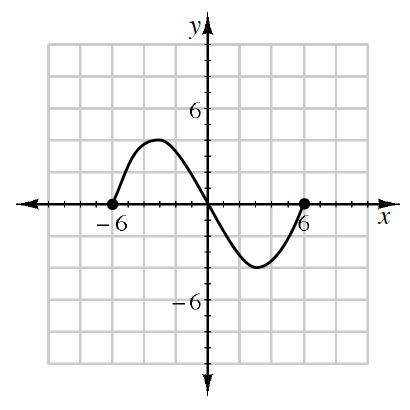### Home > INT1 > Chapter 1 > Lesson 1.2.3 > Problem1-59

1-59.

Use the relationship graphed at right to answer the questions below.

1. Is the relationship a function?

Does there appear to be more than one y-value for each value of $x$?

Yes, it is a function.

2. What is the domain?

Look at the $x$-intercepts on the graph.

$−6\le x\le6$

3. What is the range?

Look at the lowest and highest points on the graph.

$−4\le y\le4$